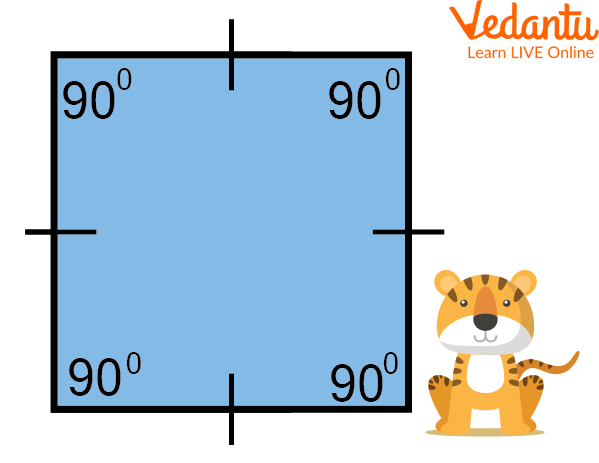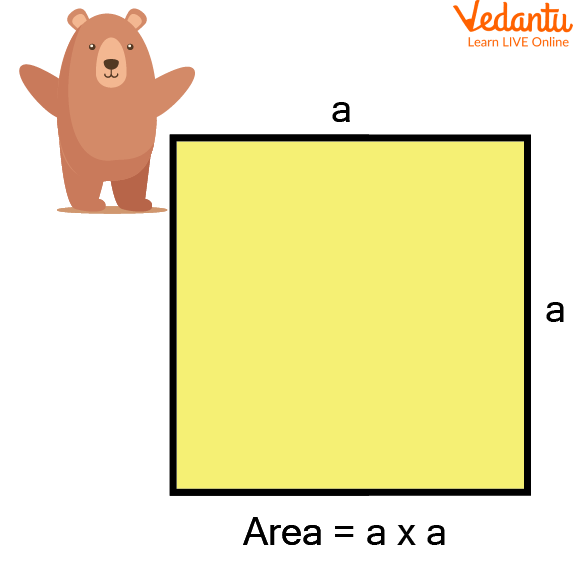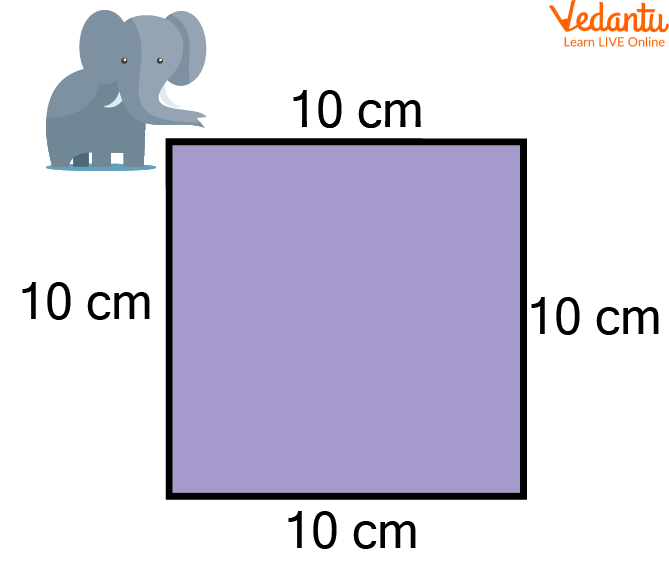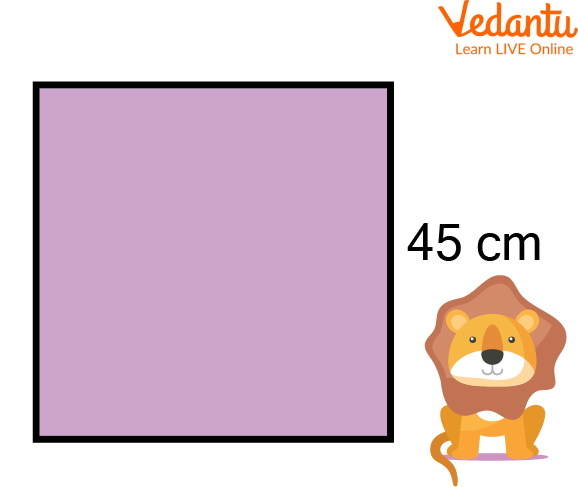Courses
Courses for Kids
Free study material
Offline Centres
More

# Area Word ProblemsLast updated date: 04th Dec 2023
Total views: 102.6k
Views today: 3.02k## Introduction to Area Word Problem

We all are aware of what squares are and how to find the area of a square. But in area word problems on the topic square, we will find the area in which the four sides are given as equal. To find the area of a given square, we must ensure that the sides of the square are in the same unit of length. In case they are given in different units, change them to the same unit. Some area of square examples is given to better understand the topic.

## What is a Square?

A regular quadrilateral in which all four sides are of equal length and all four angles are equal is considered a square. The angles of the square are 90 degrees each. Also, the square's diagonals are equal and bisect each other at 90 degrees.A Square

The above figure represents a square in which all the sides are equal, and each angle is 90 degrees.

Similarly, a parallelogram with all of its two adjacent sides being equal with one right vertex angle is a square.

## Properties of a Square

The properties of a square are listed below:

• All the interior angles are equal to 90°

• All the sides of the square are equal and congruent with each other

• The opposite sides of the square are always parallel to each other

• The diagonal of the square divides it into similar isosceles triangles

• The length of diagonals is always greater than the sides of the square

• The square’s diagonals bisect each other at 90°

• Both diagonals of the square are equal to each other

• The square contains 4 vertices and 4 sides

## Area of Square Formula

Since we know that a square is a shape which has four equal sides and every angle is a right angle, i.e. 90°. And hence, the opposite sides are also parallel. So the area of the square can be found by measuring the space occupied within the square. The formula to calculate the square of its side gives it.

If ‘a’ is the side of the square, then its area is given by ${a}^2$.Area of a Square

## Solved Word Problems on the Area of a Square

Some Solved Word Problems on the Area of a Square are given below:

Q 1. Find the area of a square whose length is 10 cm.

Ans: Given the length of a square = 10 cmSquare of 10 cm

Area of a square $=$ length $\times$ length

$=10 \times 10 \mathrm{~cm}^2$

$=100 \mathrm{~cm}^2$

Thus, the area of the square is $100 \mathrm{~cm}^2$

Q 2. Find the area of a square whose side measures 45 cm.

Ans: Given the length of a square = 45 cmSquare of 45 cm

Area of a square $=$ length $\times$ length

$=45 \times 45 \mathrm{~cm}^2$

$=2025 \mathrm{~cm}^2$

Thus, the area of the square is $2025 \mathrm{~cm}^2$.

Q 3. The length of a side of a square field is 200 m. What will be the cost of levelling the field at a rate of 10 rs per square metre?

Ans: Length of the square field = 200 m

Area of the field $=$ side $\times$ side

$=200 \mathrm{~m} \times 200 \mathrm{~m}$

$=40000 \mathrm{~m}^2$

Cost of levelling the field $=40000 \times 10 \mathrm{Rs}$

$=400000 \mathrm{Rs}$

Thus, the cost of levelling the field is 400000 Rs.

## Practice Problems on the Area of a Square

Try the given practice problems on the area of a square:

Q 1. Find the area of the square whose sides are given below:

(i) 15 m

(ii) 250 m

(iii) 5 cm

(iv) 40 cm

(v) 10 m

Ans: (i) $225 \mathrm{~m}^2$

(ii) $62500 \mathrm{~m}^2$

(iii) $25 \mathrm{~cm}^2$

(iv) $1600 \mathrm{~cm}^2$

(v) $100 \mathrm{~m}^2$

Q 2. Find the area of the square of side $16 \mathrm{~cm}$.

Ans: $256 \mathrm{~cm}^2$

Q 3. Find the length of the square whose area is $100 \mathrm{~cm}^2$.

Ans: 10 cm

## Summary

In this article, we learned about a quadrilateral square with four equal sides and all the angles as right angles, i.e. 90 degrees. The square's diagonals are equal and bisect each other at 90 degrees. We also learned about the various properties of squares and how we can calculate the area of squares. We also discussed the area of square examples to get to know how to find the area of a square of a given length easily.

## FAQs on Area Word Problems

1. Can a rhombus be considered a square?

A rhombus can also be considered a square if it follows some conditions. Rhombus is a convex quadrilateral with all four sides equal. It is said to be a square if it has a right vertex angle.

2. How is a square different from a rectangle?

A square has all its sides equal in length, whereas a rectangle has only its opposite sides equal in length. The adjacent sides are unequal in rectangles but are equal in squares.

3. Is zero a square number?

Since zero satisfies all the definitions of being a square number, it is considered a perfect square.